Cart0 items
Total price \$0
English  Русский

# Technical support

### Application of AIS technology in vessel traffic services (VTS)

Application of AIS technology in vessel traffic services (VTS).

15.12.2021

### Measuring distances and time

Distances at sea are measured in sea miles. Because the globe is slightly flattened towards the poles a latitude minute actually varies between 1841 m at the equator and 1862 m at the poles.

A sea mile has been internationally agreed upon to be 1852 meters long. In practical navigation we consider this equivalent to a latitude minute. A nautical mile is shortened to M, or sometimes nm, Nm (nautical mile).

A tenth of a nautical mile is traditionally zalled a cable length, or cable for short.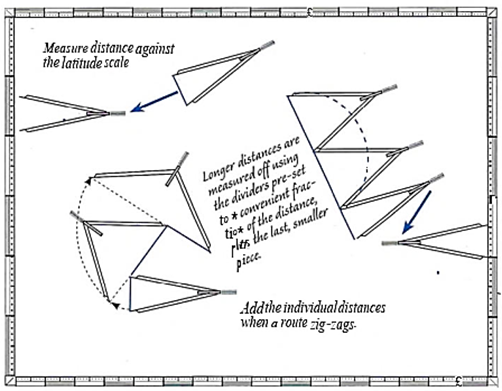Whhen measuring distances on the chart one uses the latitude scales on the left and right edges of the chart. For charts based on Mercator's projection it is good cractise to take out the measurements on the same latitude as the distance to be measured, as the scale on these charts ucreases towards the poles

Calculating speed

Sceed is distance per unit of time and at sea is measured in nodes. One knot is a speed of one nautical mile per hour

To calculate the speed - in the absence of a log, for example - one measures the time taken to traverse a known distance and then divides that by the time.

Wher situations that require speed to be calculated are; when a certain position must be reached by a given time, zrizge and lock openings, a certain position on a falling tide position. The situation can be the exact opposite - nottoarrive ZDO early for a docking, or to pick up the pilot, etc.

There are a number of terms for speed:

• Speed through water (STW) is the speed of the vessel in relation to the water surrounding the vessel. This is measured by a log.
• Speed over ground (SOG) is the speed of the vessel relative to the sea bottom. Some logs and satellite receivers can measure this, otherwise it has to be calculated. SOG is the real speed of the vessel and differs from the speed on the log when the vessel is affected by a current. Sometimes called Speed Made Good (SMG).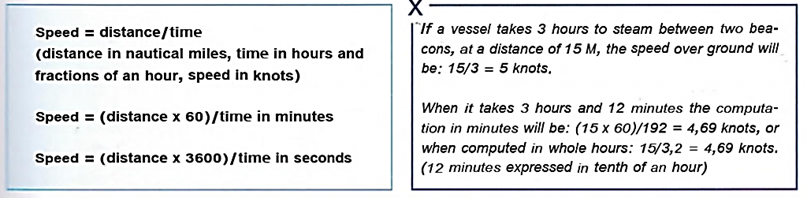Speed calculation

Different distances
Information about the speed of the vessel, enables the Navigator to answer the question "How far haveIcome (from a certain position)?", The formula used is:

Distance = speed x time in hours
Distance = (speed x time in minutes)/60

The speed can be obtained from an electronic log, a chip log, an estimate or from the knowledge that a certain rpm on the engine gives a known speed.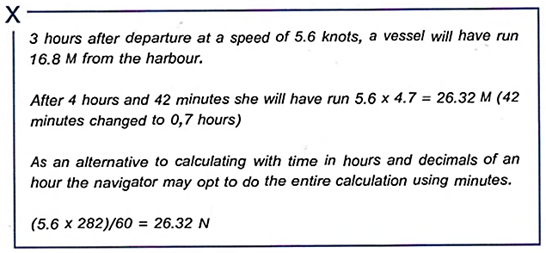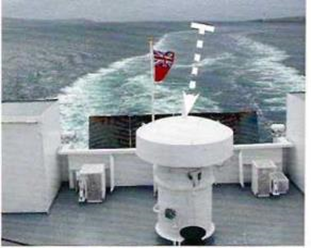Speed x time from departure gives travailed distance

Distance over ground - travelled distance measured by distance between observed positions or according to logs that measure against the sea floor.

Distance through water - travelled distance measured by any log that measure against the passing water.

Distance made good - travelled distance in the direction to a certain destination or waypoint.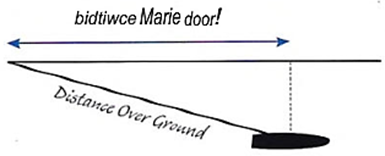Calculating time

How long will it take to reach ... at this speed?

To calculate this the Navigator needs to know the remaining distance and the speed.

Time = distance/ speed
Time in minutes = (distance/ speed)60

This question is usually met when planning a passage and the accuracy of the answer need not be very high. It is enough to use hours and minutes which are converted to one or two places of decimals.
Several expressions of time are used when planning a passage:

• ETA, estimated time of arrival
• ETD, estimated time of departure
• ATA, Actual Time of Arrival, for example when reporting to the Administration.
Provided that the need for accuracy is not great then an estimate can be  obtained by adjusting the dividers to the distance run in a certain time and then  stepping off the total length of the leg.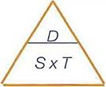Memory aid for speed, time and distance. Cover the parameter that you want and the correct formula is apparent.Simple planning - measuring time with the dividers. For example, at a speed of 6 knots a nautical miles takes ten minutes

The latitude and longitude of a given rcsition on the chart are read off jsing a pair of dividers.

The method is used to describe a rcsition, for example when in distress, when a position shall be transfmed from one chart to another or when the position shall be entered in me log book.

Positions can also be described using rearing and distance to a defined object. Sometimes this is essential!To find the position of an object on the chart, use the dividers to measure the distances to the nearest meridian and parallel. Make sure to know in which direction the values increase.

 On Chart 3471 CHARTS 1312 AND 2873: POSITIONS Positions on chart 3471 differ from those on charts 1312 and 2873 by varying amounts; positions should be transferred by bearing and distance  from common charted objects, not by latitude and longitude.

Plotting latitude and longitude
If you know the latitude and longitude of a position, for example from the GNSS receiver or from Notices to Mariners, then it can be plotted onto the chart using the following method.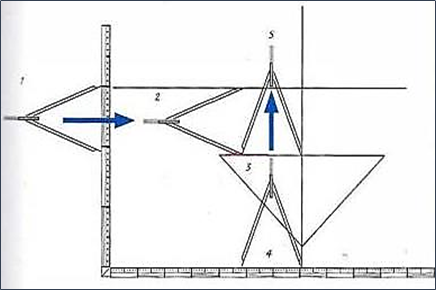Use compasses to determine the position of an object on a map. to measure the distance to the nearest meridian and parallel. Make sure you know in which direction the values ​​are increasing

Back to the list